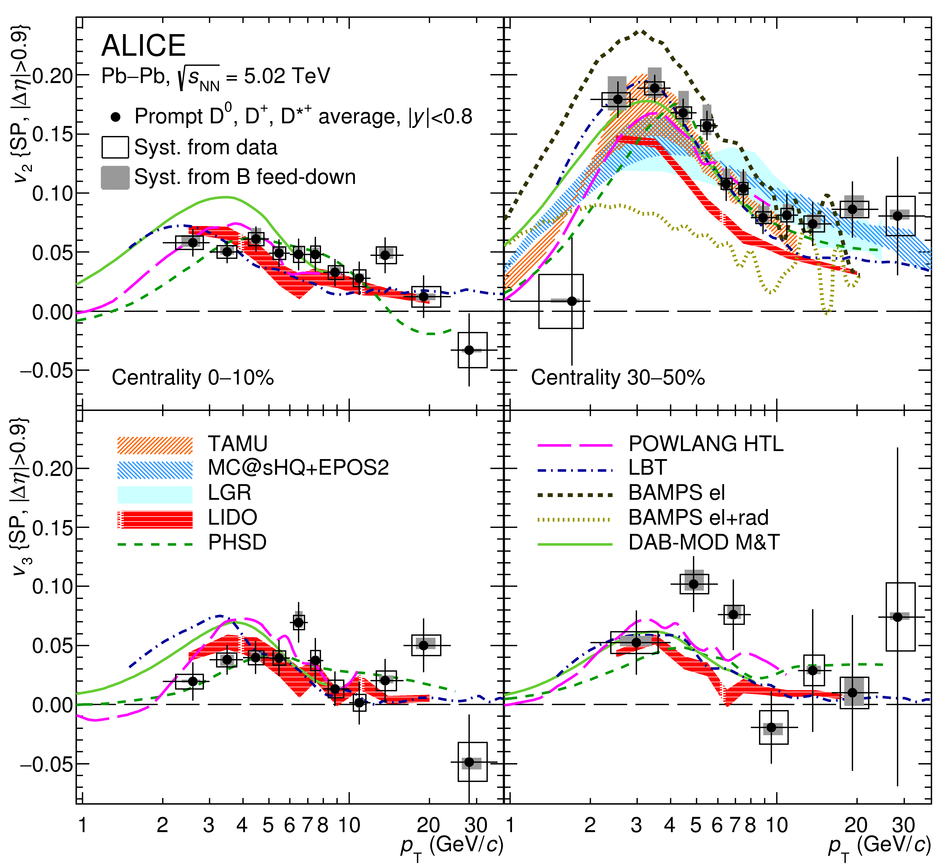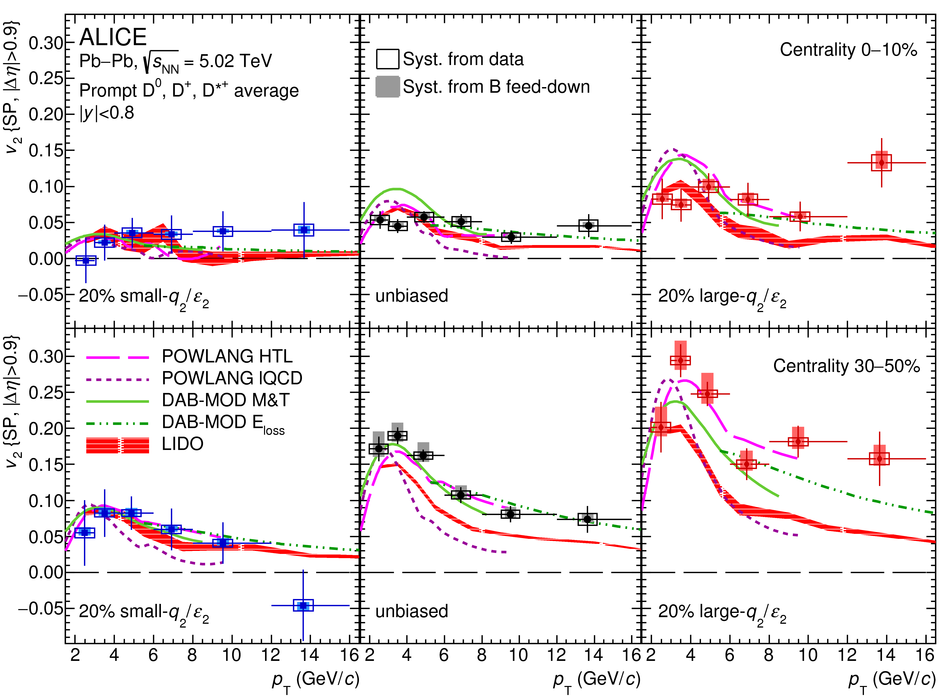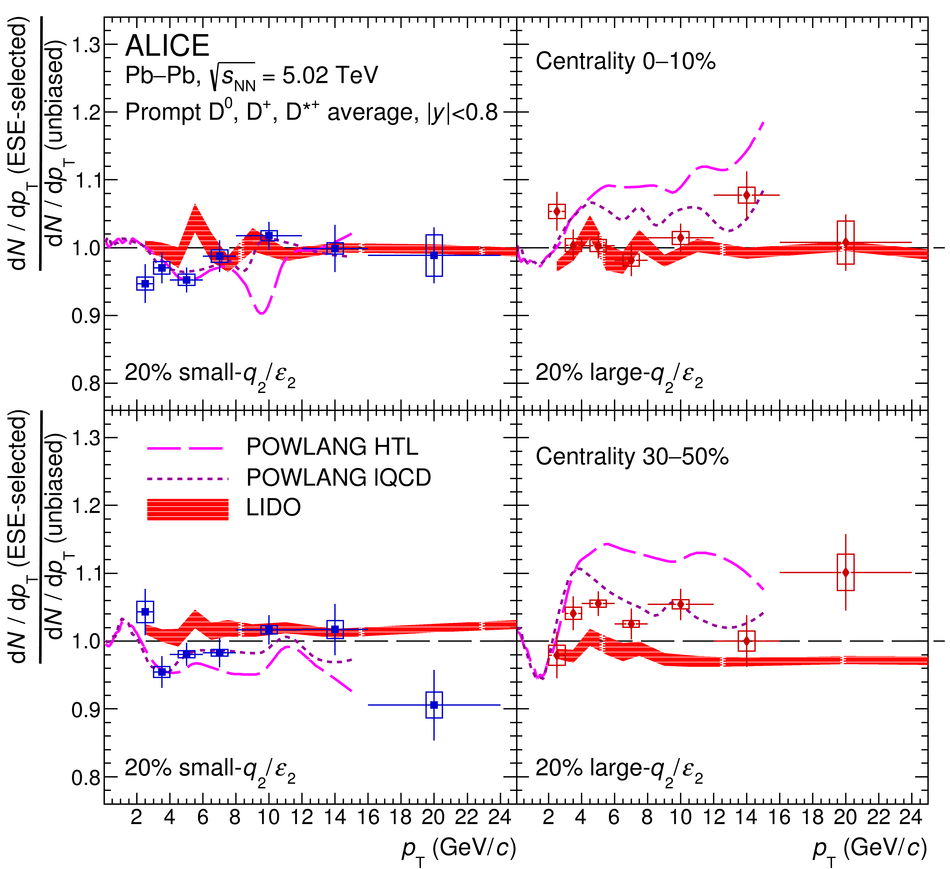# Transverse-momentum and event-shape dependence of D-meson flow harmonics in Pb-Pb collisions at $\sqrt{s_{NN}} = 5.02$ TeV

The elliptic and triangular flow coefficients $v_2$ and $v_3$ of prompt $D^{0}$, $D^{+}$, and $D^{*+}$ mesons were measured at midrapidity ($|y|<~0.8$) in Pb-Pb collisions at the centre-of-mass energy per nucleon pair of $\sqrt{s_{NN}} = 5.02$ TeV with the ALICE detector at the LHC. The D mesons were reconstructed via their hadronic decays in the transverse momentum interval $1<~ p_{\rm T}<~36$ GeV/$c$ in central (0-10%) and semi-central (30-50%) collisions. Compared to pions, protons, and J/$\psi$ mesons, the average D-meson $v_{n}$ harmonics are found to follow a mass ordering for $p_{\rm T}<~3$ GeV/$c$, and to be similar to those of charged pions for higher $p_{\rm T}$. The coupling of the charm quark to the light quarks in the underlying medium is further investigated with the application of the event-shape engineering (ESE) technique to the D-meson $v_2$ and $p_{\rm T}$-differential yields. The D-meson $v_2$ is correlated with average bulk elliptic flow in both central and semi-central collisions. Within the current precision, the ratios of per-event D-meson yields in the ESE-selected and unbiased samples are found to be compatible with unity. All the measurements are found to be reasonably well described by theoretical calculations including the effects of charm-quark transport and the recombination of charm quarks with light quarks in a hydrodynamically expanding medium.

Submitted to: PLB
HEP Data
e-Print: arXiv:2005.11131 | PDF | inSPIRE
CERN-EP-2020-082

Figures

## Figure 1

 Simultaneous fits to the invariant-mass spectrum and $v_2$ ($M_{\rm{D}}$) of $\Dzero$ (left panel), $\Dplus$ (middle panel), and $\Dstar$ (right panel) meson candidates in the $3< \pt< 4~\GeV/c$, $5< \pt< 6~\GeV/c$, and $8< \pt< 10~\GeV/c$ intervals, respectively, for the 30--50\% centrality class. The solid blue and the dotted red curves represent the total and the combinatorial-background fit functions, respectively. For the $\Dzero$ candidates, the green dashed curve represents the contribution of the reflected signal.## Figure 2

 Average $v_2$ (top panels) and $v_3$ (bottom panels) coefficients of prompt $\Dzero$, $\Dplus$, and $\Dstar$ mesons as a function of $\pt$ for $\PbPb$ collisions at $\sqrtsNN=5.02~\TeV$ in the 0--10\% (left panels) and 30--50\% (right panels) centrality classes. The $v_2$ and $v_3$ of $\pi^\pm$, $\ppbar$~ and inclusive $\Jpsi$ mesons~ measured at the same centre-of-mass energy and in the same centrality classes are shown for comparison.## Figure 3

 Average $v_2$ (top panels) and $v_3$ (bottom panels) coefficients of prompt $\Dzero$, $\Dplus$, and $\Dstar$ mesons as a function of $\pt$ for $\PbPb$ collisions at $\sqrtsNN=5.02~\TeV$ in the 0--10\% (left panels) and 30--50\% (right panels) centrality classes compared with model calculations~.## Figure 4

 Average of prompt $\Dzero$, $\Dplus$, and $\Dstar$ meson $v_2$ as a function of $\pt$ in $\PbPb$ collisions at $\sqrtsNN=5.02~\TeV$ in the small-$q_2$, large-$q_2$ (see text for details), and unbiased samples, for the 0--10\% (top panels) and 30--50\% (bottom panels) centrality classes, compared to model calculations~. In the \lido{} and \dabmod{} predictions, the ESE selection is performed with a $q_2$ estimator, while in the \powlang{} model the elliptic eccentricity $\epsilon_2$ is used.## Figure 5

 Ratio of the average prompt $\Dzero$, $\Dplus$, and $\Dstar$ meson $v_2$ coefficients measured in the small-$q_2$ (left panels) and large-$q_2$ (right panels) selected samples with respect to that of the unbiased sample as a function of $\pt$ in $\PbPb$ collisions at $\sqrtsNN=5.02~\TeV$ for the 0--10\% (top panels) and 30--50\% (bottom panels) centrality classes, compared to model calculations~.## Figure 6

 Average of the ratio of $\pt$-differential $\Dzero$, $\Dplus$, and $\Dstar$ yields measured in the ESE-selected samples to those in the unbiased sample in $\PbPb$ collisions at $\sqrtsNN=5.02~\TeV$ for the 0--10\% (top panels) and 30--50\% (bottom panels) centrality classes, compared to the \powlang~ and \lido~ predictions.